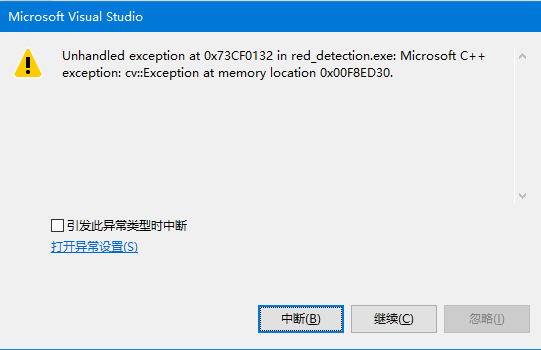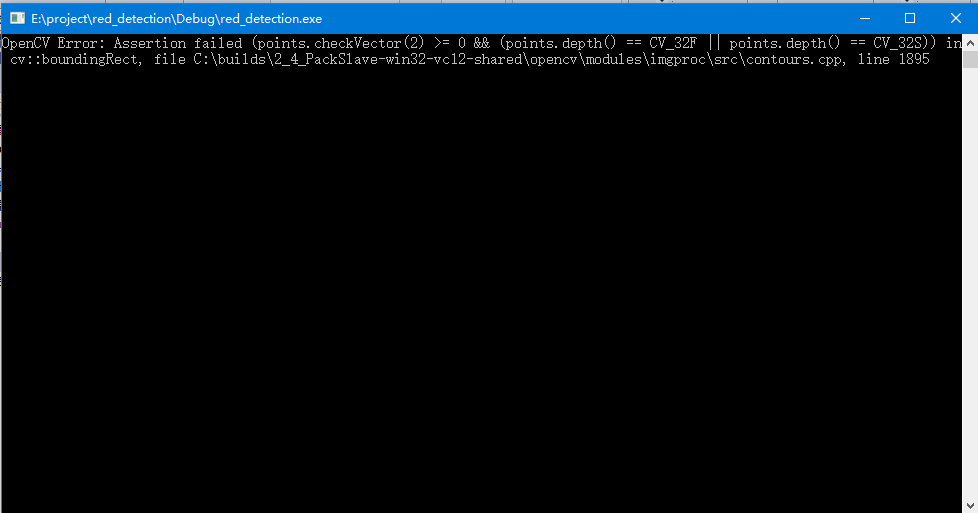opencv用boundingrect找最大区域时崩溃 40C

//#include "stdafx.h"

#include "opencv2/opencv.hpp"

#include

#include
using namespace std;
using namespace cv;

//漫水填充
void fillHole(const Mat srcBw, Mat &dstBw)
{
Size m_Size = srcBw.size();
Mat Temp = Mat::zeros(m_Size.height + 2, m_Size.width + 2, srcBw.type());//延展图像
srcBw.copyTo(Temp(Range(1, m_Size.height + 1), Range(1, m_Size.width + 1)));

``````cv::floodFill(Temp, Point(0, 0), Scalar(255,255,255));
//cv::floodFill(Temp, Point(30, 29), Scalar(255, 0, 0), 0, Scalar(10, 10, 10), Scalar(10, 10, 10));
Mat cutImg;//裁剪延展的图像
Temp(Range(1, m_Size.height + 1), Range(1, m_Size.width + 1)).copyTo(cutImg);

dstBw = srcBw | (~cutImg);
``````

}

int main(int argc, char** argv)
{
//定义扫描图像的循环变量
int i = 0;
int j = 0;

``````//通过摄像头采集视频

VideoCapture capture(0);

//读取视频.
//VideoCapture capture("E:/weixian.mp4");
//视频总帧数
//num是一个计数的flag.
int num = 1;

while (1)
{
//IplImage* frame;
Mat frame1, frame;
//将捕获图像存入frame变量
capture >> frame1;

blur(frame1, frame1, Size(7, 7));

//缩放倍数
double fScale = 0.5;
//目标图像尺寸
CvSize czSize;
czSize.width = frame1.cols*fScale;
czSize.height = frame1.rows*fScale;
cv::resize(frame1, frame, cv::Size(czSize.width, czSize.height), (0, 0), (0, 0), cv::INTER_LINEAR);
cv::Mat rgbImage = frame, hsvImage;
//转换到hsv空间
cv::cvtColor(rgbImage, hsvImage, cv::COLOR_BGR2HSV);
//为了获取图像的尺寸
//这里把图像类型转换
IplImage* tempImage = &IplImage(hsvImage);
IplImage* extractionImage = &IplImage(hsvImage);
IplImage* reversionImage = &IplImage(hsvImage);
IplImage* grayImage = &IplImage(hsvImage);
IplImage* binaryImage = &IplImage(hsvImage);
//显示两个空间下的图像
imshow("RGB", rgbImage);
//imshow("HSV", hsvImage);
for (i = 0; i < tempImage->height; i++)
{
for (j = 0; j < tempImage->width; j++)
{
//获取像素点为（j, i）点的HSV的值
CvScalar s_hsv = cvGet2D(tempImage, i, j);
/*
opencv 的H范围是0~180，红色的H范围大概是(0~8)∪(160,180)
S是饱和度，一般是大于一个值,S过低就是灰色（参考值S>80)，
V是亮度，过低就是黑色，过高就是白色(参考值220>V>50)。
*/
CvScalar s;
if (!(((s_hsv.val>0) && (s_hsv.val<8)) || (s_hsv.val>178) && (s_hsv.val<180)))
{
s.val = 0;
s.val = 0;
s.val = 0;
cvSet2D(tempImage, i, j, s);
}

//else这小段是自己加的，如果是红色，就置为白色.
else
{
s.val = 180;
s.val = 30;
s.val = 255;
cvSet2D(tempImage, i, j, s);
}
}
}
//提取红色分量
cvConvert(tempImage, extractionImage);
//cvNamedWindow("Extraction");
//cvShowImage("Extraction", extractionImage);
//颜色空间变换回RGB
cvCvtColor(extractionImage, reversionImage, cv::COLOR_HSV2BGR);
//cvNamedWindow("Reversion");
//cvShowImage("Reversion", reversionImage);
//这里如果转灰度,会出现内存泄漏.
//由于前边定义每个图像都用的取址运算符
//因为grayImge指向的是hsvImage的地址,而后者一直在变
//所以此处gray也一直在变
//RGB图像转灰度图
//cvCvtColor(reversionImage, grayImage, cv::COLOR_BGR2GRAY);
//cvNamedWindow("Gray");
//cvShowImage("Gray", grayImage);
//灰度图转二值图
cvThreshold(grayImage, binaryImage, 100, 255, CV_THRESH_BINARY);
//cvThreshold(reversionImage, binaryImage, 150, 255, CV_THRESH_BINARY);
//cvNamedWindow("Binary");
//cvShowImage("Binary", binaryImage);
//5*5正方形，8位uchar型，全1结构元素
cv::Mat element5(5, 5, CV_8U, cv::Scalar(1));
cv::Mat closed,opened,temp,final,cimage;
//cv::vector<vector<cv::Point>> contours(10000);
temp = Mat(binaryImage);
//高级形态学运算函数
cv::morphologyEx(temp, opened, cv::MORPH_CLOSE, element5);
cv::morphologyEx(temp, closed, cv::MORPH_CLOSE, element5);
//cvNamedWindow("Opened");
//imshow("Opened", opened);
//cvNamedWindow("Closed");
//imshow("Closed", closed);
fillHole(closed, final);
cvNamedWindow("Final");
imshow("Final", final);
Canny(final, cimage, 150, 250);
//cvNamedWindow("Canny");
//imshow("Canny", cimage);

cv::vector<vector<cv::Point>> contours;
vector<Vec4i> hierarchy;

cv::findContours(cimage, contours, CV_RETR_EXTERNAL, CV_CHAIN_APPROX_NONE,Point());
//cv::findContours(cimage, contours, hierarchy, RETR_TREE, CHAIN_APPROX_SIMPLE, Point());

// 寻找最大连通域

double maxArea = 0;
vector<cv::Point> maxContour;
for (size_t i = 0; i < contours.size(); i++)
{
double area = cv::contourArea(contours[i]);
if (area > maxArea)
{
maxArea = area;
maxContour = contours[i];
}

//cout << maxArea << endl;
}
//cout << maxArea << endl;
//cout << contours.size() << endl;
//cout << i << endl;

//cout << maxContour << endl;

// 将轮廓转为矩形框

cv::Rect maxRect = cv::boundingRect(maxContour);

// 显示连通域
cv::Mat result1, result2;
final.copyTo(result1);
final.copyTo(result2);

for (size_t i = 0; i < contours.size(); i++)
{
cv::Rect r = cv::boundingRect(contours[i]);
cv::rectangle(result1, r, cv::Scalar(255));
}
//cv::imshow("all regions", result1);
//cv::waitKey();
cv::rectangle(result2, maxRect, cv::Scalar(0, 255, 0), 3);
cv::imshow("largest region", result2);
if (maxArea>8000)
{
cv::imshow("largest region", result2);
}

/*

*/

waitKey(20);

//视频帧数加1.
num = num + 1;
}
return 0;
``````

}

``````
``````2个回答cxz1988 您好！请问这个问题原因是什么呢？您有解决方案吗

`````` Mat conMat(contours[i].size(), 2, CV_32FC1);
for(int i = 0; i < contours[i].size(); i ++)
{
conMat.at<float>(i, 0) = contours[i].x;
conMat.at<float>(i, 1) = contours[i].y;
}
cv::Rect maxRect = cv::boundingRect(conMat);
``````opencv　提取一个连通区域内的颜色

opencv　提取一个连通区域内的颜色，是不是通过提取连通分量就可以了,例如一个装菜的碟子，提取碟子的颜色;图像形态学了解比较少，多谢

opencv imshow()崩溃 急求救

openCV 标示感兴趣区域的外轮廓

OpenCV 怎样提取类似方形的区域

opencv圆环形感兴区域设置

opencv找不到dll的问题

opencv 2.4.9 ,VS2010中用debug模式可以正常运行，但用release模式就崩溃

Opencv如何发现图像内部指定区域与周围的相似度？

opencv+c++怎么实现对目标区域的粗定位

QtCreator5.3.1使用opencv显示图像，**crash

android开发中使用opencv，如何解决内存泄漏问题？

OPENCV对摄像头ROI区域设定的问题

C语言下使用OpenCV接口崩溃问题求助

opencv里面找不到opencv_createsamples.exe如何训练一个分类器

opencv里面找不到opencv_createsamples.exe如何训练一个分类器 现在想自己训练一个分类器但是没有opencv_createsamples.exe都不知道怎么办了

Java基础知识面试题（2020最新版）

Intellij IDEA 实用插件安利

1. 前言从2020 年 JVM 生态报告解读 可以看出Intellij IDEA 目前已经稳坐 Java IDE 头把交椅。而且统计得出付费用户已经超过了八成（国外统计）。IDEA 的...

MySQL数据库面试题（2020最新版）

2020阿里全球数学大赛：3万名高手、4道题、2天2夜未交卷

HashMap底层实现原理，红黑树，B+树，B树的结构原理 Spring的AOP和IOC是什么？它们常见的使用场景有哪些？Spring事务，事务的属性，传播行为，数据库隔离级别 Spring和SpringMVC，MyBatis以及SpringBoot的注解分别有哪些？SpringMVC的工作原理，SpringBoot框架的优点，MyBatis框架的优点 SpringCould组件有哪些，他们...

《Oracle Java SE编程自学与面试指南》最佳学习路线图2020年最新版（进大厂必备）